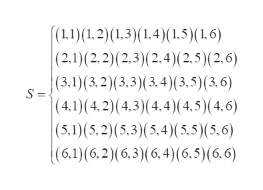# A pair of dice is rolled 60 times and the sums are recorded. Determine the empirical probability of rolling a sum of 2.

Question
181 views

A pair of dice is rolled 60 times and the sums are recorded. Determine the empirical probability of rolling a sum of 2.

check_circle

Step 1

To find that the empirical probability of rolling a sum of 2 (two).

Definition of Empirical probability:

The empirical probability of an event E is given by, the ratio of the number of times the event E has occurred to the total number of times the experiment has been performed.

Step 2

Here,

Let E denotes the event that a sum of two appears on the pair of dice,

If the pair of dice is rolled, sample space ishelp_outlineImage Transcriptionclose(L1)(1.2)(1.3)(1,4)(1,5)(1.6) (2.1)(2,2)(2.3)(2.4)(2,5) (2, 6) (3,1)(3,2) (3,3)(3,4)(3,5) (3, 6) |(4.1)(4,2) (4,3)(4.4)(4,5)(4, 6) (5,1)(5,2)(5,3)(5.4)(5,5)(5,6) (6,1)(6,2) (6,3)(6,4) (6,5)(6,6) S = fullscreen
Step 3

The pair to get sum of 2 is (1, 2).

The numbe...

### Want to see the full answer?

See Solution

#### Want to see this answer and more?

Solutions are written by subject experts who are available 24/7. Questions are typically answered within 1 hour.*

See Solution
*Response times may vary by subject and question.
Tagged in

### Basic Probability